# Hardy inequality

(diff) ← Older revision | Latest revision (diff) | Newer revision → (diff)

for series

If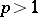,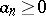and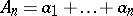,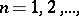then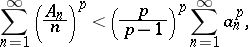except when all the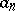are zero. The constant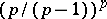in this inequality is best possible.

The Hardy inequalities for integrals are:and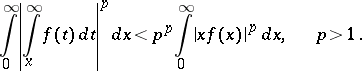The inequalities are valid for all functions for which the right-hand sides are finite, except whenvanishes almost-everywhere on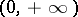. (In this case the inequalities turn into equalities.) The constantsand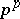are best possible.

The integral Hardy inequalities can be generalized to arbitrary intervals: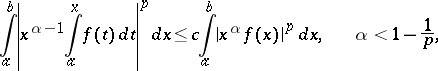where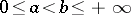,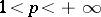, and where the's are certain constants.

Generalized Hardy inequalities are inequalities of the form(1)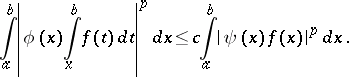(2)

If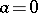and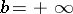, inequality (1) holds if and only if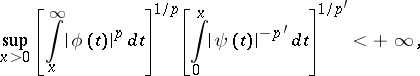and (2) holds if and only if